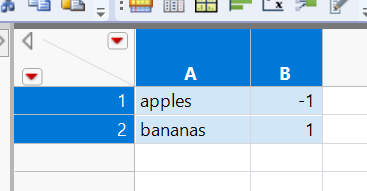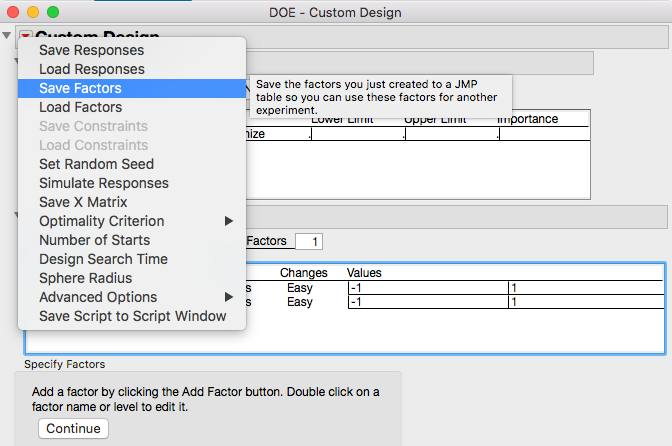Choose Language Hide Translation Bar
Highlighted

## Saving a data table as a factors table

Good Afternoon,

Is there a way with either GUI or jsl to save an ordinary data table as a factors table?

For example:Kind Regards,

Ben

1 ACCEPTED SOLUTION

Accepted Solutions
Highlighted

## Re: Saving a data table as a factors table

Thanks for your answers Jim and Ian, I don't think I added enough detail to my question. I basically have a data table of a lot of factors (and their low/high, categorical values) that I want to get into a DOE dialog and don't wish to go through one by one and put them in the DOE.

The code below worked (Jim you'll notice a code snippet from another question you answered). After doing this script, going into the DOE and using "Load Factors" populates with the required information.

``````dt1 = Data Table("FullDoE_factors"); //Many factors in this table but need design role

nomdata = dt1 << get column names( nominal );
condata = dt1 << get column names( continuous );

For( i = 1, i <= N Items( nomdata ), i++,
Column( dt1, Char( nomdata[i] ) ) << Set Property("Design Role", Design Role( Categorical ))
);

For( i = 1, i <= N Items( condata ), i++,
Column( dt1, Char( condata[i] ) ) << Set Property("Design Role", Design Role( Continuous ))
);``````

5 REPLIES 5
Highlighted

## Re: Saving a data table as a factors table

Here is the script from a Saved Factors table.  It should give you a clue as to what it takes to make a Factors Table.

``````New Table( "zippy",
New Table Variable( "Table Type", "DOE Factor Table" ),
New Column( "X1",
Numeric,
"Continuous",
Format( "Best", 12 ),
Set Property( "Design Role", Design Role( Continuous ) ),
Set Property( "Factor Changes", Easy ),
Set Selected,
Set Values( [-1, 1] )
),
New Column( "X2",
Numeric,
"Continuous",
Format( "Best", 12 ),
Set Property( "Design Role", Design Role( Discrete Numeric ) ),
Set Property( "Factor Changes", Easy ),
Set Selected,
Set Values( [-1, 1] )
)
)``````
Jim
Highlighted

## Re: Saving a data table as a factors table

Probably you know this already, but if you use the GUI to get things the way you want, you can then 'Save Factors' to make the table you are after:If you then want to use JSL, you can always get the script to regenerate the resulting table from 'Copy Table Script' from the red triangle. You then paste the clipboard into a new script window, and proceed from there . . .

Highlighted

## Re: Saving a data table as a factors table

Thanks for your answers Jim and Ian, I don't think I added enough detail to my question. I basically have a data table of a lot of factors (and their low/high, categorical values) that I want to get into a DOE dialog and don't wish to go through one by one and put them in the DOE.

The code below worked (Jim you'll notice a code snippet from another question you answered). After doing this script, going into the DOE and using "Load Factors" populates with the required information.

``````dt1 = Data Table("FullDoE_factors"); //Many factors in this table but need design role

nomdata = dt1 << get column names( nominal );
condata = dt1 << get column names( continuous );

For( i = 1, i <= N Items( nomdata ), i++,
Column( dt1, Char( nomdata[i] ) ) << Set Property("Design Role", Design Role( Categorical ))
);

For( i = 1, i <= N Items( condata ), i++,
Column( dt1, Char( condata[i] ) ) << Set Property("Design Role", Design Role( Continuous ))
);``````

Highlighted

## Re: Saving a data table as a factors table

Is there a question?  Or are you indicating that you have your solution?

Jim
Highlighted

## Re: Saving a data table as a factors table

Hi Jim, I have my solution and have now marked as such. Thanks for your time.

Kind Regards,

Ben

Article Labels

There are no labels assigned to this post.